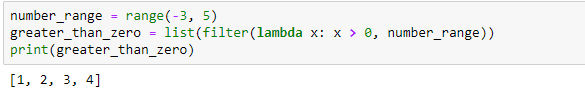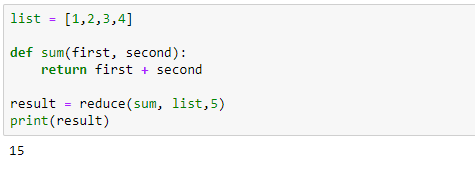# Filter and Reduce Functions

## Introduction

As discussed in the previous tutorial on Map functions, Filter, and Reduce are typical examples of functional programming. They allow us to write simpler, shorter code, without bothering about loops and branching.

Let us see how to use these functions in this tutorial.

## Filter Function

Filter function returns boolean values (which is : true or false) and then passes each element in the iterable through the function, "filtering" away those that are false or we can say that it creates a list of elements for which a function returns true.

The filter() has the following syntax:

`filter(function, iterable)`

Only one iterable is required for the filter functions where it passes each element in the iterable through the function and returns only the ones that evaluate to true.

Let’s take a look at an example to understand how filter works:

Below is a list of the ages of 10 random people.We want to check ages for people who are above the age of 35.

Using lambda with filter function:

In the below example, we are checking if a word is palindrome or not. A “palindrome” is a word that reads the same forward as backward.

Another example :Finding numbers greater than Zero in a given range of numbers

## Reduce Function

The reduce() function has the following syntax:

`reduce(function, iterable[,initial])`

Where function is the function on which each element in the iterable gets applied to, initial is the (optional) value that gets placed before the elements of the iterable in the calculation, and serves as a default when the iterable is empty. Reduce applies a function of two arguments cumulatively to the elements of an iterable, optionally starting with an initial argument. It is a useful function for performing computation on a list and returning the result.

Let’s take a look at an example to understand how reduce works:

We want to find the sum of list of integers. The traditional way is using a for loop:

Now, let’s try with reduce:

In this eg., reduce takes the first and second elements in the list and passes it to the function sum where it computes their sum and returns it to reduce which then takes that result and applies it as the first element to sum and takes the next element (third) in the list as the second element to sum. It does this continuously (cumulatively) until entire list is exhausted.

What if we use the optional initial value:Here, reduce uses 5(initial value) as the first argument to sum.

Using lambda with reduce function:

The same above example(finding sum) using lambda function with reduce.

## Conclusion

In this tutorial, with the help of few examples we learnt how to use the filter and reduce functions in python.

--

--

--

## More from The Startup

Get smarter at building your thing. Follow to join The Startup’s +8 million monthly readers & +756K followers.

## How does it know?! Some beginner chatbot tech for newbies.## How to use Jira## AZURE CLOUD PLATFORM -Azure Database For MySQL Server (Article 11)## Introducing Synthetic Real Estate## Use Python and The Number Insights API to Analyze Leads## Creating an environment with Airflow and DBT on AWS (part 1)## Using Nginx Ingress Controller to Manage HTTP/1.1 and HTTP/2 Protocols## The secrets of Agoda’s uptime — A day in the life of a NOC engineerData Scientist

## Some tips for using fixtures in pytest## Interview Tips: How to use Lists efficiently in Python## Tech-Travel Time